Aptitude Tests 4 Me

Non Verbal Logic

Detailed solution

57.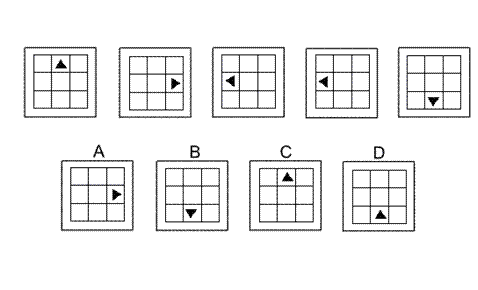Solution: B Explanation: In this question the black triangle is reflected successively in three axes of symmetry: 1. The diagonal stretching from the bottom left corner of the grid to the top right corner. 2. The vertical line through the centre of the grid. 3. The horizontal line through the centre of the grid. Following these rules, to obtain the next diagram in the sequence, the next reflection should be type 2. The correct answer, therefore, is B.

58.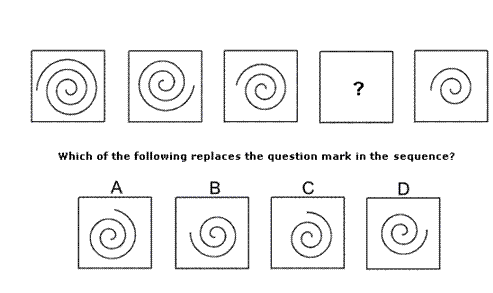Solution: D Explanation: In this question, there are two rules to follow. The first rule is that a 90° arc is erased every time from the outer extremity of the spiral. Following this rule, the correct answer could be B, C or D. The second rule is that the remaining part of the spiral is rotated by 90° clockwise each time. Following this rule, the correct answer must be D.

59.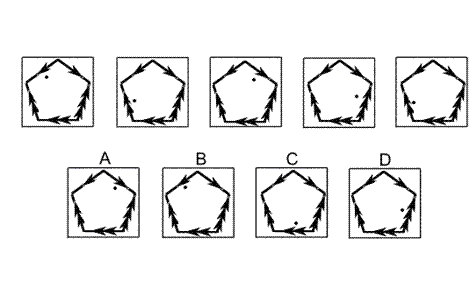Solution: A Explanation: In this question the dot rotates around the inside of the pentagon. The direction of the arrow from its previous position indicates whether the rotation is clockwise or anticlockwise. The number of arrowheads on the arrow of its previous position indicates the number of fifths of a complete turn by which it should rotate. For the next diagram in the sequence, therefore, it should rotate two fifths of a complete turn in a clockwise sense. The correct answer, therefore, is A.

60.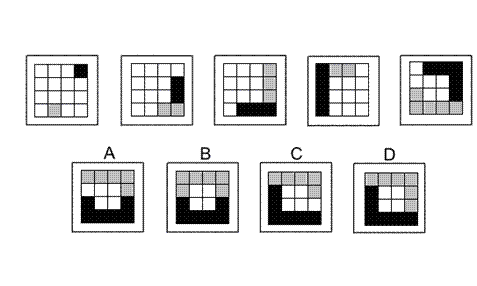Solution: D Explanation: In this question the black squares move around the perimeter of the grid in a clockwise sense and the grey square move around the perimeter of the grid in an anticlockwise sense. From one diagram to the next, the number of black squares increases by one each time and the number of grey squares also increases by one each time. At the same time, the longer train of squares occupies the next available squares around the perimeter of the grid as they move around (black, clockwise and grey anticlockwise). Where black and grey squares overlap, only the black is visible. Following these rules, the next diagram of the sequence must be D

61.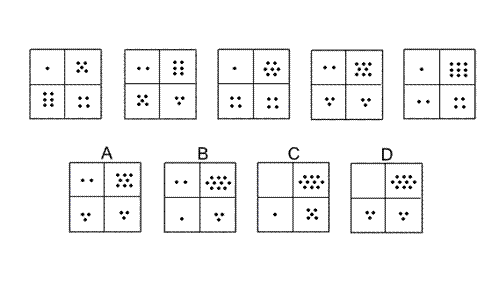Solution: B Explanation: In this question there are two rules to follow that are applied successively. The first rule is that the numbers of dots in the top row increase by one while the numbers of dots in the second row decrease by one. The second rule is that the numbers of dots in the first column decrease by one while the numbers of dots in the second column increase by one. The next rule to follow, therefore, is the first rule. The correct answer, therefore, is B.

62.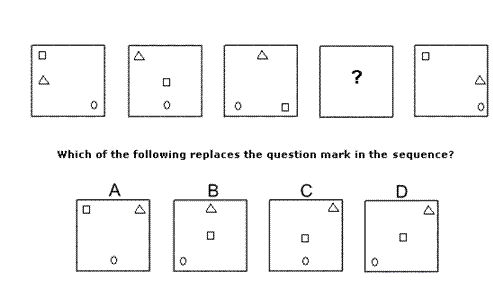Solution: C Explanation: In this question, each of the three shapes has its own rule to follow. The triangle rotates clockwise around the perimeter of the square occupying eight positions, moving one place each time. Following this rule, for the missing diagram of the sequence, the triangle should be in the top right corner of the square. The correct answer, therefore, could be A, C or D. The small square occupies the three positions on the main diagonal of the large square that stretches from the top left corner to the bottom right corner. It also moves one place each time and keeps going backwards and forwards along the diagonal. Following, this rule, for the missing diagram of the sequence, the small square should be in the centre of the large square. The correct answer, therefore, could be C or D. The circle moves along the bottom edge of the square, occupying three positions one place at a time, and keeps going backwards and forwards along the bottom edge. Following this rule, for the missing diagram of the sequence, the circle should be in the centre of the bottom edge. The correct answer, therefore, is C.

63.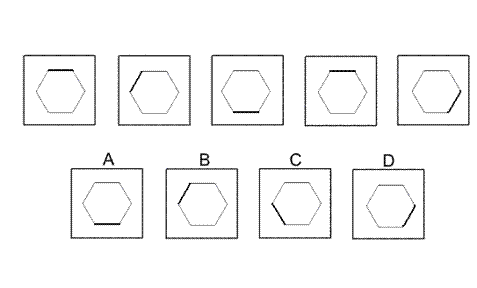Solution: A Explanation: In this question there is a regular hexagon with one edge shaded darker that the others. The ruleis that the hexagon rotates anticlockwise about its centre point by multiples of 60° - the first time by 60°, the second by 2 × 60° = 120°, the third by 3 × 60° = 180° etc. Following this rule, to obtain the next diagram in the sequence, the hexagon should rotate by 5 × 60° = 300° anticlockwise. The correct answer, therefore, is A.

64.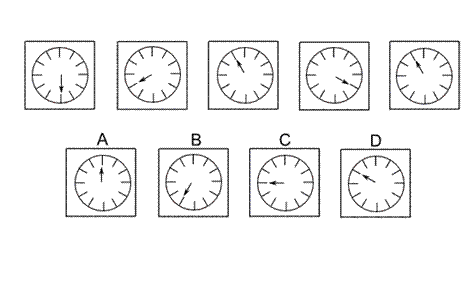Solution: D Explanation: In this question, the hand rotates clockwise around the clock face. The numbers of places it rotates successively are the numbers of the sequence of prime numbers - 2, 3, 5, 7 etc. The next prime number in the sequence is 11, so the next diagram in the sequence must be D (where the hand of the clock has progressed by 11 places clockwise).

65.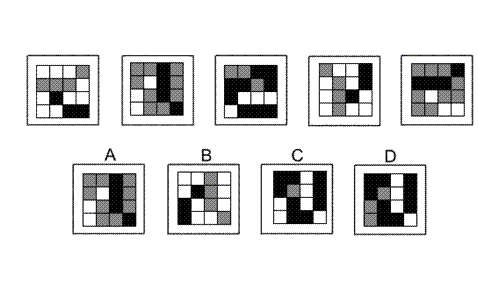Solution: D Explanation: In this question there are two rules to follow that must be applied at the same time. The first rule is that the grid should be rotated by 90° clockwise each time. The second rule is that the colours of the squares change according to the rule - black changes to white, white changes to grey and grey changes to black. When these two rules are applied at the same time, then the next diagram in the sequence must be D.

66.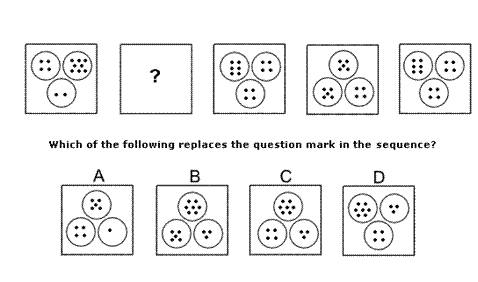Solution: C Explanation: In this question there are two rules to follow. The first rule concerns the circles which rotate by 60° anticlockwise each time. Following this rule, the missing diagram of the sequence could be A, B or C. The second rule concerns the number of dots. The circle with four dots always has four dots. The circle that starts with two dots increases by one dot each time. The circle that starts with eight dots decreases by one each time. Following this rule, the missing diagram of the sequence must be C.

67.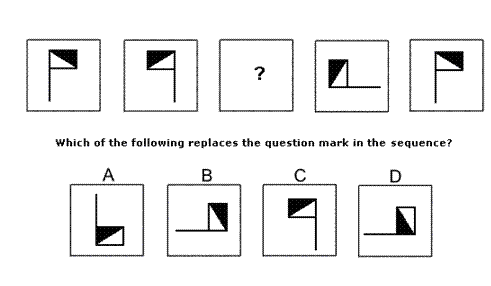Solution: B Explanation: In this question there are two rules to follow that are applied one after the other. The first rule is that the flag is reflected in the vertical axis. The second rule is that the flag is rotated by 90° clockwise. To find the missing diagram of the sequence, the second rule should be applied. The correct answer, therefore, is B.

 1 2 3 4 5 6 7 8 9 10 11 12 13 14 15 16 17 18 19 20 21 22 23 24 25 26 27 28 29 30 31 32 33 34 35 36 37 38 39 40 41 42 43 44 45 46 47 48 49 50 51 52 53 54 55 56 57 58 59 60 61 62 63 64 65 66 67 68 69 70 71 72 73 74 75 76 77 78 79 80 81 82 83 84 85 86 87 88Passage ReadingVerbal LogicNon Verbal LogicNumerical LogicData InterpretationReasoningAnalytical AbilityBasic NumeracyAbout UsContactPrivacy PolicyMajor TestsFAQ